## Laser hazard distance equations

This page presents laser safety equations and example calculations. These are valid for the type of lasers commonly misused by the general public: laser pointers and commercially available handheld lasers. Specifically, this means lasers emitting visible light (400-700 nanometers) that is continuous (e.g., not pulsed lasers).

Also, these equations assume an unwanted exposure where a person would move and/or close their eyes within 1/4 second of seeing the bright light. This is a standard assumption in the laser safety field. The Maximum Permissible Exposure value for a 1/4 second exposure is therefore applicable. (Injuries have occurred where a person deliberately stared into a laser beam for much longer than 1/4 second. See this list of self-inflicted laser eye injuries for some examples.)

The equations and examples on this page are color coded. Pink represents the power in milliwatts, purple represents the beam divergence in milliradians, and brown represents the Visual Correction Factor. The color coding helps you see where to plug in these values into the equations.

The online Laser Hazard Distance Calculator uses the equations below. The inputs to the calculator also follow the pink-purple-brown color coding.

#### NOHD and visual interference distance equations

It is easy to calculate the eye hazard distance (NOHD), and the three visual interference distances corresponding to flashblindness, glare and distraction. All you need to know is the laser’s power in milliwatts, the beam divergence in milliradians, and the beam color (from which we determine the Visual Correction Factor).

If you don’t know the beam divergence, use 1 milliradian for lasers under 500 milliwatts in power, and 1.5 milliradians for lasers 500 milliwatts and above. Here are the equations, which are explained in much more detail below.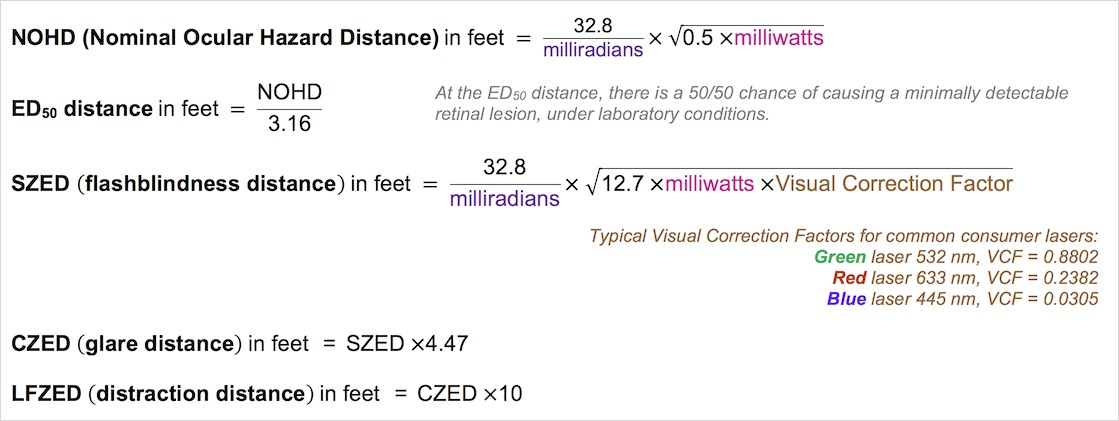These calculations make some simplifying assumptions which are valid for continuous-wave visible lasers at aircraft distances. The SZED differs from the formula found in FAA Advisory Circular 70-1 by converting watts to milliwatts. This in turn makes the constant under the square root to be “12.7” and not the “1.27” found in AC 70-1

Let’s find out what the NOHD and visual interference distances mean, and then look at some detailed examples.

## Background information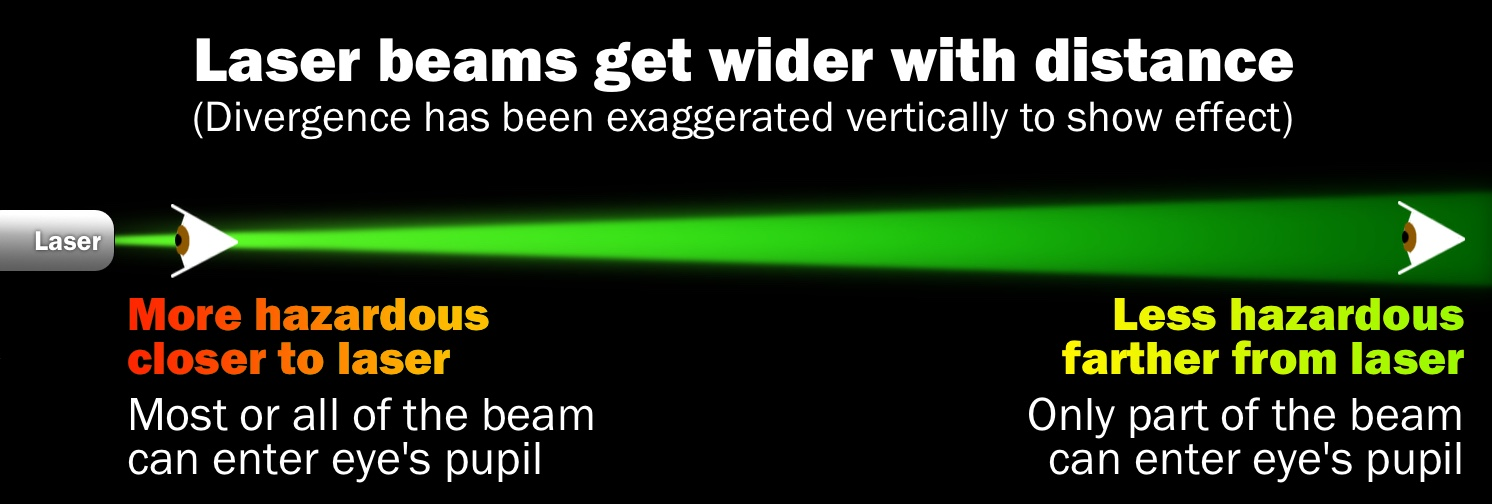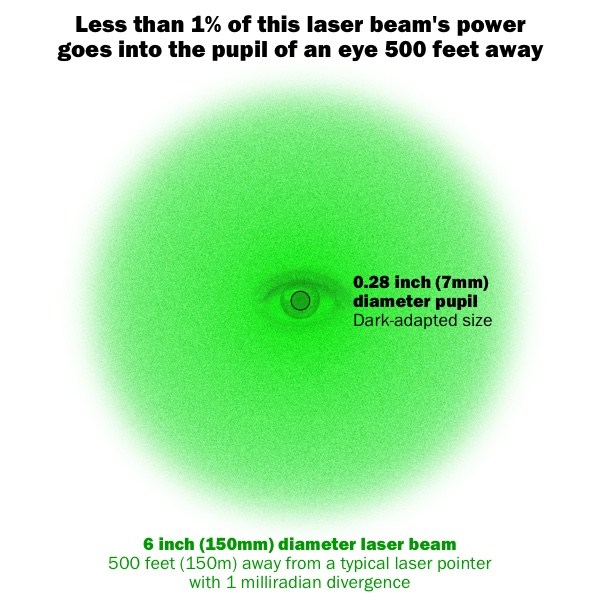#### What are the NOHD and the ED50 distances?

For pilots, an important concept is the Nominal Ocular Hazard Distance. Laser safety experts recommend not having direct eye exposure to a laser’s beam closer than the NOHD for that laser. That’s because the beam’s power density (irradiance) from the laser source to the NOHD exceeds the Maximum Permissible Exposure limit set by scientists of 2.54 milliwatts per square centimeter. Once beyond the NOHD, the beam is considered completely eye safe since the irradiance falls below the MPE limit.

However, this does not mean that an eye exposure within the NOHD will automatically cause an eye injury, or even is likely to cause an injury. The NOHD is a “nominal” hazard distance, not an actual hazard distance.

[Technical details: The ANSI Z136.1 laser safety standard sets the Maximum Permissible Exposure to be “a factor of 10 below the 50% damage level.” Exposure at the MPE gives “…a negligible probability of damage.” For visible light (and near infrared light) “the MPE is well below the exposure required to produce a minimal (or threshold) lesion. For purposes of this standard, a minimal retinal lesion is the smallest ophthalmoscopically visible change in the retina. This change is a small white patch (apparently coagulation that occurs within 24 hours of the time of exposure.)]

The location along a beam path where the exposure is 10 times the MPE is at 31.6% of the NOHD. This means that at a distance of 31.6% of the NOHD, there is a 50/50 chance that an exposure will cause a minimally detectable change to the retina. We call this the ED50 (Exposure Dose 50%) distance. The diagram below color-codes a laser’s eye hazard, so red is a definite hazard, yellow is a potential hazard, and green is not considered to be hazardous.The NOHD and ED50 distance concepts are shown below for three typical lasers.Click on the chart to see it larger.

For all lasers of this type, the ED50 distance (marked “50/50” on the chart) is 31.6% of the Nominal Ocular Hazard Distance. As you can see from the yellow-green and green areas, the NOHD concept includes a built-in “safety margin.”

#### What are the visual interference distances?

The FAA is not only concerned with pilots’ eye safety. They also are concerned with safe but too-bright lasers. Effects such as temporary flashblindness, glare and distraction can interfere with pilots’ vision and performance.

The FAA has set three zones around airports, and has set limits on the power density (irradiance) of lasers within these zones.

• Sensitive Zone Exposure Distance: The beam is bright enough to cause temporary vision impairment, from the source to this distance. Beyond this distance, the beam is 100μW/cm^2 or less (below 100 microwatts per square centimeter).
• Critical Zone Exposure Distance: The beam is bright enough to cause a distraction interfering with critical task performance, from the source to this distance. Beyond this distance, the beam is 5 μW/cm^2 or less (below 5 microwatts per square centimeter).
• “Laser-Free” Exposure Distance: Beyond this distance, the beam is dim enough that it is not expected to cause a distraction. This light level is 50 nW/cm^2 or less (below 50 nanowatts or 0.5 microwatts per square centimeter).The SZED is generally taken to be the flashblindness distance, the CZED is used as the glare distance, and the LFED is considered to be the distraction distance.#### Comparing NOHD and visual interference distances

This bar chart shows the NOHD (black) and visual interference distances (red, orange and yellow) for two 800 mW, 1.5 mrad lasers. They both have the same NOHD since the output power is the same. However, one laser emits green light. The human eye is more sensitive to green, so it is a visual hazard at a greater distance than an equivalent blue laser.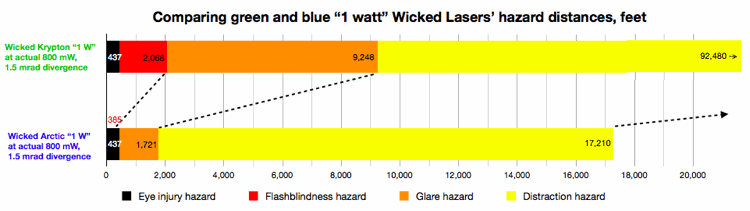For the blue laser, the flashblindness hazard (385 ft) is shorter than the NOHD (437 ft). This is due to the deep blue light not being sensed well by the eye’s retina. All of the laser power is going onto the retina -- that’s why both the green and blue lasers have the same NOHD of 437 ft. But the blue light is not seen well, so it can only flashblind up to 385 ft. In this case, the NOHD is more important. A person getting the direct beam in their eye should be farther than 437 ft, if possible.

## NOHD equations and examples in depth

#### What you need to know

To calculate the NOHD, you need to know the laser’s power in milliwatts (mW) and the beam divergence in milliradians (mrad). For NOHD calculations, the wavelength (color) does not matter.

• If you don’t know the beam divergence, use a value of 1 milliradian for lasers under 500 mW, and 1.5 milliradians for lasers 500 mW and above.
• If you know the power in watts, multiply by 1000 to get the power in milliwatts. For example, a 1 watt laser is the same as 1000 mW; a 1.5 watt laser is the same as 1500 mW.

#### NOHD and ED50 equations

The NOHD equation below is derived from FAA’s Advisory Circular 70-1, which in turn derived it from an equation in the ANSI Z136.1 laser safety standard. The ANSI equation was re-expressed by the FAA in a simpler form and to put the answer in feet. The equation makes some simplifying assumptions which are valid for continuous-wave visible lasers at aircraft distances. Use this equation ONLY for visible continuous-wave lasers (not pulsed lasers).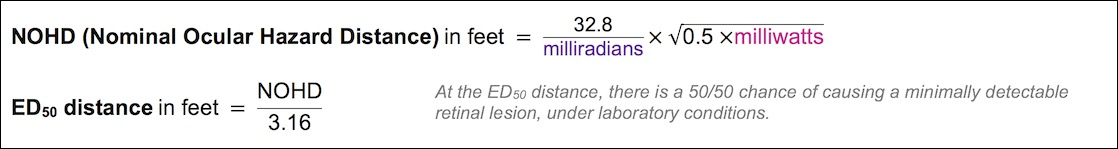Here are the same equations written out in text (not in mathematical form):

• NOHD (Nominal Ocular Hazard Distance) in feet = (32.8 ÷ milliradians) (square root of (0.5 milliwatts))
• ED50 distance in feet = NOHD ÷ 3.16. Definition: At the ED50 distance there is a 50/50 chance of causing a minimally detectable retinal lesion, under laboratory conditions.

#### NOHD examples

EXAMPLE 1: In the U.S., lasers sold as pointers must be less than 5 mW. A typical divergence is 1 milliradian. What is the Nominal Ocular Hazard Distance? The 50/50 injury chance distance?

• NOHD (Nominal Ocular Hazard Distance) in feet = (32.8 ÷ 1) (square root of (0.5 5)) = 32.8 (square root of 2.5) = 32.8 1.58 = 51.9 feet
• ED50 distance in feet = 51.9 ÷ 3.16 = 16.4 feet

Answer: The Nominal Ocular Hazard Distance of a 5 mW laser pointer with 1 mrad divergence is 51.9 feet. The ED50 distance means that if a person is 16.4 feet from the laser and is exposed under laboratory conditions (the laser and eye are fixed relative to each other), there is a 50/50 chance of causing a minimally detectable retinal lesion.

EXAMPLE 2a: One of the most controversial lasers is the “Spyder III Arctic” made by the company Wicked Lasers. This is sold as a 1 watt laser. The manufacturer says the beam divergence is 1.5 milliradians. What are the NOHD and the ED50 distances?

Note: Be sure to convert 1 watt into 1000 milliwatts, since the equation requires milliwatts.

• NOHD (Nominal Ocular Hazard Distance) in feet = (32.8 ÷ 1.5) (square root of (0.5 1000)) = 21.8667 (square root of 500) = 21.8667 22.36 = 489.0 feet
• ED50 distance in feet = 489.0 ÷ 3.16 = 154.7 feet

EXAMPLE 2b: While the Spyder III Arctic is claimed to be 1 watt, the actual measured power is in the 700 to 800 mW range. If an Arctic’s output is actually 800 mW, how does that affect the NOHD and ED50 distances?

• NOHD (Nominal Ocular Hazard Distance) in feet = (32.8 ÷ 1.5) (square root of (0.5 800)) = 21.8667 (square root of 400) = 21.8667 20 = 437.3 feet
• ED50 distance in feet = 437.3 ÷ 3.16 = 138.4 feet

Answer: It reduces the NOHD by 51.7 feet and the ED50 distance by 16.3 feet. Although the reduction in power is 20% (200 mW less power compared to 1000 mW), the reduction in NOHD is 10.5% (51.7 feet out of 489 feet). The ED50 distance is also reduced by 10.6% (16.3 feet out of 154.7 feet).

EXAMPLE 3: A laser light show uses a 40 watt laser with a 1.5 mrad beam divergence. What are the NOHD and ED50 distances?

• NOHD (Nominal Ocular Hazard Distance) in feet = (32.8 ÷ 1.5) (square root of (0.5 40000)) = 21.8667 (square root of 20000) = 21.8667 141.421 = 3092.4 feet
• ED50 distance in feet = 3092.4 ÷ 3.16 = 978.6 feet

## Visual interference equations and examples in depth

#### What you need to know

To calculate the visual interference distances, you need to know the laser’s power in milliwatts, the beam divergence in milliradians, and the color (from which we determine the Visual Correction Factor).

#### Visual interference equations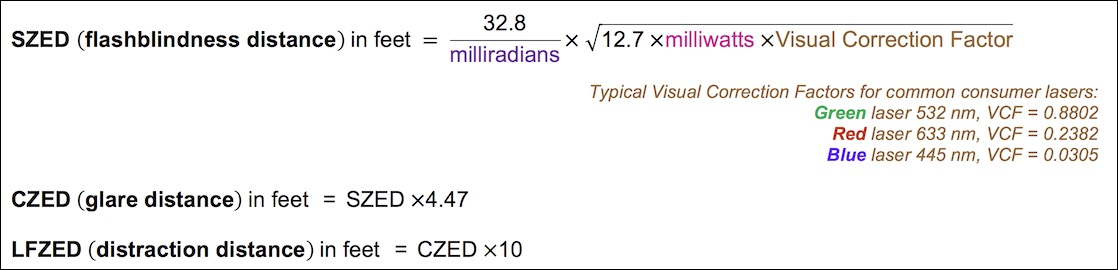Here are the same equations written out in text (not in mathematical form):

• SZED (flashblindness distance) in feet = (32.8 ÷ milliradians) (square root of (12.7 milliwatts VCF))
• CZED (glare distance) in feet = SZED 4.47
• LFZED (distraction distance) in feet = CZED 10 (this is the same as SZED 44.7)

#### Visual Correction Factors

Use the wavelength information below to determine the Visual Correction Factor, or VCF. This is done because the human eye has a different sensitivity to certain colors. We see green light as much brighter than an equivalent amount of red or blue light. The VCF takes this into account. Here are the FAA’s VCF values for the lasers most likely to be encountered by pilots:

• Green 532 nm, VCF = 0.8802. The majority of green laser pointers and handhelds currently (2017) sold to consumers. However, 520 nm pointers (VCF = 0.7092) are becoming more affordable and popular. If you do not know which green was used in an incident, assume 532 nm (VCF = 0.8802).
• Red 633 nm, VCF = 0.2382. Use this for most red diodes and helium-neon lasers. A 635 nm diode will have a slightly lower VCF (0.2202). If a laser is known to be red, but the exact type or wavelength is not known use a value of 0.2382.
• Blue 445 nm, VCF = 0.0305. This is a very popular blue. The original source is diodes taken from Casio “Green Slim” laser projectors. This type of diode is used in the Wicked Lasers Spyder III Arctic and similar blue handhelds.

Other wavelengths of interest:

• Green 555 nm, VCF = 1.0. Worst case -- use for ultra-conservative calculations when the wavelength is not known and you want to ensure even the brightest possible color is taken into account.
• Red 670 nm, VCF = 0.0321. A deeper red used in laser pointers, usually older or cheaper ones
• Blue 473 nm, VCF = 0.1054. A brighter blue used in some specialized pointers
• Cyan 488 nm, VCF = 0.1941. Used in argon gas lasers that were popular in older laser show projectors
• Violet 405 nm, VCF = 0.0008. A deep violet used in some specialized pointers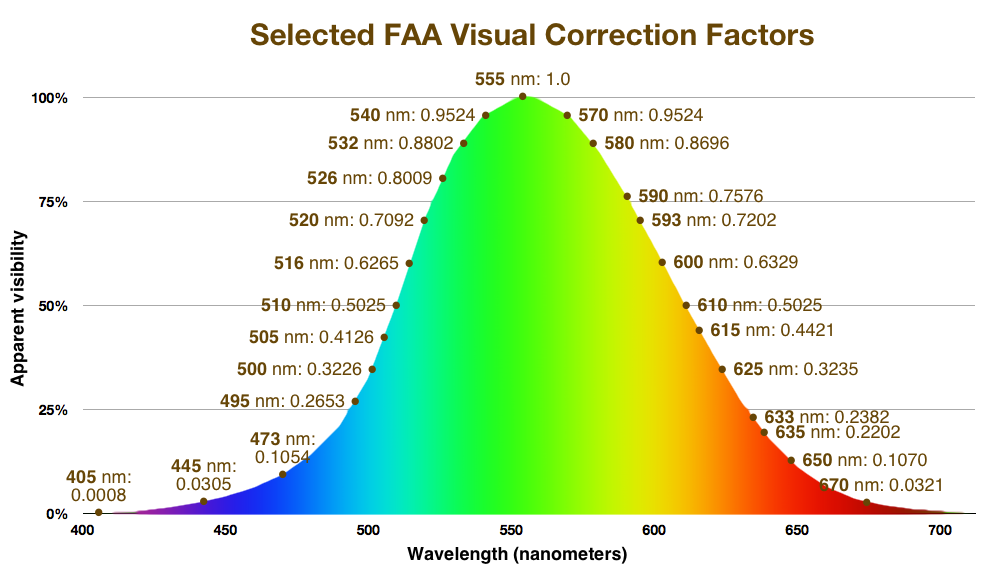#### Visual interference examples

EXAMPLE 1: What are the visual interference distances for a 5 mW green laser pointer, with 1 milliradian divergence?

For all consumer green lasers, we assume the wavelength is 532 nanometers, which has a Visual Correction Factor of 0.8802.

• SZED (flashblindness distance) in feet = (32.8 ÷ 1) (square root of (12.7 5 0.8802)) = 32.8 (square root of 55.8927) = 32.8 7.476142 = 245.21746 feet
• CZED (glare distance) in feet = 245.21746 4.47 = 1,096.12 feet
• LFZED (distraction distance) in feet = 1096.12 * 10 = 10,961.2 feet

This means that a 5 mW laser pointer could cause temporary flashblindness up to 245 feet, could cause glare up to 1,096 feet, and could be a distraction to a pilot up to a distance of 10,961 feet (2.1 miles).

EXAMPLE 2: What are the visual interference distances for the same laser, except in red?

For all consumer red lasers, if we don’t know the wavelength we use the worst-case common red which is 633 nanometers. This has a Visual Correction Factor of 0.2382.

• SZED (flashblindness distance) in feet = (32.8 ÷ 1) (square root of (12.7 5 0.2382)) = 32.8 (square root of 15.1257) = 32.8 3.8892 = 127.5658 feet
• CZED (glare distance) in feet = 127.5658 4.47 = 570.22 feet
• LFZED (distraction distance) in feet = 570.22 10 = 5,702.2 feet

The green laser is 3.6952 times more visible (compare the VCFs of 0.8802 and 0.2382). However the green laser’s visual interference distances are only 1.922 times longer than the red laser’s (compare the LFED of 10,961.2 feet with 5,702.2 feet). In fact the difference is exactly the square root of 3.6952 (1.922 is the square root of 3.6952).

This shows how if you have two equivalent lasers, except one is a different color, the brighter laser’s visual interference distance will be greater by the square root of the difference in the Visual Correction Factors.

EXAMPLE 3: Compare the NOHD and visual interference distances for a Spyder III Arctic laser. Use the actual output power of 800 mW and the manufacturer’s divergence of 1.5 milliradians.

We previously calculated the NOHD of this laser as being 437.3 feet. Here is the calculation again:

• NOHD (Nominal Ocular Hazard Distance) in feet = (32.8 ÷ 1.5) (square root of (0.5 800)) = 21.8667 (square root of 400) = 21.8667 20 = 437.3 feet

Recall that the NOHD does NOT depend on the wavelength. However, we do need to know the wavelength to determine the visual interference distances. From Wicked Lasers’ website, we see that the Arctic model emits blue light with a wavelength of 445 nanometers. This has a Visual Correction Factor of 0.0305.

• SZED (flashblindness distance) in feet = (32.8 ÷ 1.5) (square root of (12.7 800 0.0305)) = 21.8667 (square root of 309.88) = 21.8667 17.6034 = 384.9283 feet
• CZED (glare distance) in feet = 384.9283 4.47 = 1720.63 feet
• LFZED (distraction distance) in feet = 1720.63 10 = 17,206.3 feet

Notice that for this laser, the flashblindness distance of 384.9 feet is actually less than the eye hazard distance (NOHD) of 437.3 feet. This is because the human eye sees blue light poorly -- in this case, only 3% as well as the brightest green light. Although the laser may not appear dazzlingly bright, it still could be an eye hazard to a person within the NOHD. For this reason, you should not allow access within the SZED — the person would still be within the NOHD. You must set the SZED to be the same as the NOHD. (If you were filling out the FAA Advisory Circular 70-1 “Laser Configuration Worksheet,” you would be required to enter “Less than NOHD” in the SZED space.)

Here are two free laser safety calculation resources:

Easy Haz software, free online version from Kentek’s Laser Safety U. To use this, you may need to understand scientific notation. Also, results are returned in meters.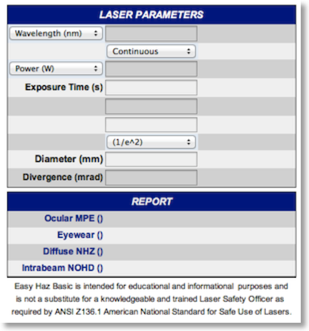Free Laser Hazard Distance safety calculator app for iPhone, iPod Touch and iPad. This app also gives the laser beam’s spot size at the NOHD. The publisher, James Stewart of LVR Limited, has additional low-cost laser safety apps available from the iOS App Store.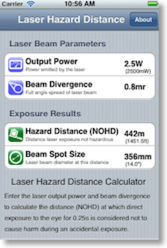Professional programs such as Eazy Haz LSO Edition and Skyzan are available for more complex situations. A search for “laser safety software” can find these programs.

For online calculations of beam diameter, beam area, and irradiance at a distance, plus a divergence calculator, see this page at laserextreme.nl.

If you are a member of the International Laser Display Association, you can use ILDA’s free online Skyzan professional laser hazard calculator. Here is a sample ILDA Skyzan report for a multi-color (RGB) laser light show taking into account light haze in the atmosphere and restricted beam angles (click screenshot to enlarge):